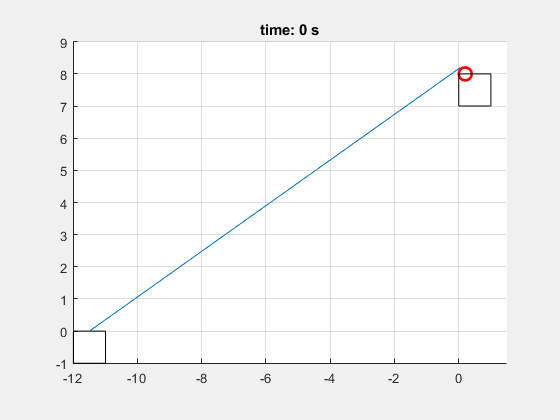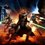# I made a Matlab physics animation

Here's a cool pulley simulation I made visualised on Matlab:I'm learning to animate simulations on Matlab. So, I decided to animate one of my coolest problems, Simulating Dynamics-2

The actual simulation was done with the help of Karan Chatrath's code solution to my problem. I modified the code to make it more efficient and added some extra features, and more importantly writing the second part of the code, which was animating the data.

The setup above is basically two masses connected by a pulley, with the second mass dragging the first mass with the help of gravity. There is friction between the first mass and the floor.

The equations of motion can be derived using Newton's Laws, here's the system in matrix form:

$\begin{bmatrix} m_1 & 0 & \mu & \cos(\theta) \\ 0 & m_2 & 0 & 1 \\ 0 & 0 & 1 & \sin(\theta)\\ 2x & 28-2y & 0 & 0 \end{bmatrix}$ $\begin{bmatrix} \ddot{x} \\ \ddot{y} \\ N \\ T \end{bmatrix} = \begin{bmatrix} 0 \\ m_2 g \\ m_1g \\ 2\dot{x}^2 + 2\dot{y}^2 \end{bmatrix}$

We can solve for these values simply by multiplying the inverse system matrix by the vector on the right hand side. Once we do this, we have to numerically integrate $\ddot{x},\ddot{y}$, the first and second indices of the solution to $A^{-1}b$.

For the animation, just draw two squares with the tops at the position of the point masses at x coordinate for the first mass $x$ and y coordinate for the second mass $y$. Then draw lines from the hinge to each mass.

To maintain accuracy, I added a counter to add the values of time, $x$, and (y) to arrays. The animation works by while looping through these arrays, drawing the data, deleting the data, and redrawing through the loops.

The values I used for the simulation are $m_1 = 3, m_2 = 2, g = 10, \mu = 0.8, l = 14m$

  1 2 3 4 5 6 7 8 9 10 11 12 13 14 15 16 17 18 19 20 21 22 23 24 25 26 27 28 29 30 31 32 33 34 35 36 37 38 39 40 41 42 43 44 45 46 47 48 49 50 51 52 53 54 55 56 57 58 59 60 61 62 63 64 65 66 67 68 69 70 71 72 73 74 75 76 77 78 79 80 81 82 83 84 85 86 87 88 89 90 91 92 93 94 95 96 97 98 99 100 101 102 103 104 105 106 107 108 109 110 111 clear all; close all; clc; %% Initialisation and constants deltaT= 10^-5; time = 0; m1 = 3; m2 = 2; g = 10; mu = 0.8; x = -14*cos(asin(8/14)); y = 0; xDot = 0; yDot = 0; massPosition1 = []; massPosition2 = []; times = []; count = 0; %% Simulation while xDot >= 0 if mod(count, 1000) == 0 times = [times; time]; massPosition1 = [massPosition1; x]; massPosition2 = [massPosition2; y]; end %System matrix A = [m1 0 mu -x/(y-14);0 m2 0 1;0 0 1 (1 - x^2/(y - 14)^2)^(1/2);2*x 28-2*y 0 0]; %Vector b = [0;m2*g;m1*g;2*yDot^2-2*xDot^2]; %Solution vector (accelerations, tension, and normal force) S = inv(A)*b; xDotDot = S(1); yDotDot = S(2); %Numerical Integration xDot = xDot + xDotDot*deltaT; yDot = yDot + yDotDot*deltaT; x = x + xDot*deltaT; y = y + yDot*deltaT; %Updating time and counter time = time + deltaT; count = count + 1; end %% Plot axis(gca,"equal"); O = [0 0]; Hinge = [0.2 8]; axis([-12 1.5 -1 9]); grid on; %% Animation i = 1; while i <= length(times) %animation speed control conditional statement if mod(i,5) == 0 %Hinge circle hingeCircle = viscircles(Hinge, 0.2); %time title("time: " + num2str(ceil(times(i))) + " s"); %Positions and string line plotting Mass_1 = rectangle('Position',[(massPosition1(i)-0.5) -1 1 1]); stringToHinge1 = line([massPosition1(i) Hinge(1)-0.15],[0 Hinge(2)+0.2]); Mass_2 = rectangle('Position', [0 (7-massPosition2(i)) 1 1]); stringToHinge2 = line([0.4 0.4], [8 (8-massPosition2(i))]); %pausing for animation pause(10^-6); %Deleting previous elements to draw new ones in next iteration if i < length(times)-5 delete(Mass_1); delete(stringToHinge1); delete(Mass_2); delete(stringToHinge2); end end %looping through arrays i = i+1; end %% End 

Have a try for yourself, or, if you don't have Matlab, take a look at the GIF above.Note by Krishna Karthik
7 months ago

This discussion board is a place to discuss our Daily Challenges and the math and science related to those challenges. Explanations are more than just a solution — they should explain the steps and thinking strategies that you used to obtain the solution. Comments should further the discussion of math and science.

When posting on Brilliant:

• Use the emojis to react to an explanation, whether you're congratulating a job well done , or just really confused .
• Ask specific questions about the challenge or the steps in somebody's explanation. Well-posed questions can add a lot to the discussion, but posting "I don't understand!" doesn't help anyone.
• Try to contribute something new to the discussion, whether it is an extension, generalization or other idea related to the challenge.

MarkdownAppears as
*italics* or _italics_ italics
**bold** or __bold__ bold
- bulleted- list
• bulleted
• list
1. numbered2. list
1. numbered
2. list
Note: you must add a full line of space before and after lists for them to show up correctly
paragraph 1paragraph 2

paragraph 1

paragraph 2

[example link](https://brilliant.org)example link
> This is a quote
This is a quote
    # I indented these lines
# 4 spaces, and now they show
# up as a code block.

print "hello world"
# I indented these lines
# 4 spaces, and now they show
# up as a code block.

print "hello world"
MathAppears as
Remember to wrap math in $$ ... $$ or $ ... $ to ensure proper formatting.
2 \times 3 $2 \times 3$
2^{34} $2^{34}$
a_{i-1} $a_{i-1}$
\frac{2}{3} $\frac{2}{3}$
\sqrt{2} $\sqrt{2}$
\sum_{i=1}^3 $\sum_{i=1}^3$
\sin \theta $\sin \theta$
\boxed{123} $\boxed{123}$

Sort by:

@Percy Jackson

@NSCS 747

In case you're interested. I couldn't get Karan Chatrath or Steven Chase's tag though lol.

@Talulah Riley If you're active anymore.

- 7 months ago

Nice bro! Your mentions are all wrong. I didn't get the notif as they're not blue. I can mention them for you though - @NSCS 747, @Talulah Riley, @Karan Chatrath, @Steven Chase

- 6 months, 4 weeks ago

Yeah; I may have edited the comment. When you edit comments, all your mentions go away. Btw thanks a lot for adding them back :)

- 6 months, 3 weeks ago

no prob bro :)

- 6 months, 3 weeks ago

Impressive work

- 6 months, 3 weeks ago

Thanks. Seeing it visualised adds a level of cool to simulations.

- 6 months, 3 weeks ago